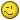# Sumproduct in matrix means

W

#### Walter Briscoe

I have some data on which I calculate row and column means.
There are discrepancies when the data is incomplete.
I have simplified the data to show the problem.

I believe it may be possible to use Sumproduct to calculate correct
results. I hope some reader can say how.

I have simple data
A B C D
1 AM PM Means
2 Tuesday 2 2
3 Monday 3 4 3.5
4 Means 2.5 4 3.25/2.75

R2C4 is =Average(B2:C2) and copied down
R4C2 is =Average(B2:B3) and copied across
R4C4 shows the row and column means, and is =Average(B4:C4) & "/" &
Average(D23)

The true value for R4C4 should be 3/3
3 is the result of =Average(B2:C3) and is one way of getting true
values. I want to use row and column means also to produce a true value.
3 is also the result of (R4C2*2+R4C3*1)/3
3 is also the result of (R2C4*1+R3C4*2)/3
=sumproduct(B4:C4,[count(B2:B3),count(C2:C3)])/count(B2:C3)
is roughly what I want in place of =Average(B4:C4).

I could use auxiliary cells in sumproduct but would prefer not to do so.

That is not quite right.My true data refers to 7 days and 4 time periods -
blood sugars for a week.J

#### joeu2004

Walter Briscoe said:
I have simple data
A B C D
1 AM PM Means
2 Tuesday 2 2
3 Monday 3 4 3.5
4 Means 2.5 4 3.25/2.75

R2C4 is =Average(B2:C2) and copied down
R4C2 is =Average(B2:B3) and copied across
R4C4 shows the row and column means, and is =Average(B4:C4) & "/" &
Average(D23)
The true value for R4C4 should be 3/3

(Why complicate things by oscillating between R1C1 and A1 reference styles?
Rhetorical question. The point is: don't!)

The real problem is with your math: the average of averages is not
necessarily the same as the average of all. They are equal only with the
divisors of each average are the same, which is not true in your example.

Column D is the average daily number. Row 4 is the average AM or PM number
across days.

If you want D4 to be the overall average, it should be written as
=AVERAGE(B2:C3).

J

#### joeu2004

PS.... I wrote said:
The real problem is with your math: the average of averages is not
necessarily the same as the average of all. They are equal only with the
divisors of each average are the same, which is not true in your example.

Column D is the average daily number. Row 4 is the average AM or PM
number across days.

If you want D4 to be the overall average, it should be written as
=AVERAGE(B2:C3).

After reading your original posting in more detail, I see that you
understand that to some degree.

The alternative method is: multiply each average by the number of items
averaged (the original divisor), add all of those products, and divide by
the total number of items (the sum of the original divisors).

Without translating that into a proper format -- it can be done, but it
seems unnecessary -- let me just comment this way....

Walter Briscoe said:
=sumproduct(B4:C4,[count(B2:B3),count(C2:C3)])/count(B2:C3)
is roughly what I want in place of =Average(B4:C4).

Yes, that is close to the correct alternative conceptually.

But I don't see the point of it since, without helper cells, you must do
COUNT(B2:C3) as you noted. And that is no less trouble than AVERAGE(B2:C3).
In fact, the whole thing is a lot more trouble.

"Everything should be made as simple as possible, but not simpler." --
Albert Einstein

J

#### joeu2004

Minor errata (nitpicking)....
The real problem is with your math: the average of averages
is not necessarily the same as the average of all. They are
equal only [when] the divisors of each average are the same,
which is not true in your example.

I shouldn't say that is the "only" time they are equal. Of course, there
are examples when they are equal by coincidence (e.g. when all the numbers
to be averaged are equal). And there are examples when they are "close
enough" to equal; for example, equal to 1 decimal place.